# Exploring the Mandelbrot set

March 3, 20210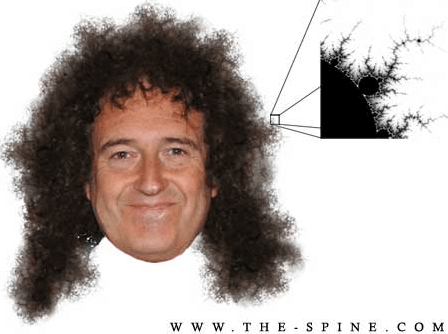#### Introduction

In March 1980, a supercomputer at the IBM Research Centre in York Heights, New York, gave instructions to an ancient Tektronix printing device. The device diligently dotted a blank sheet of paper in interesting places, and when it finally stopped clacking, the result looked as if a handful of dust had been thrown onto the page. Benoit Mandelbrot could not believe his eyes. He was clearly aware that he was witnessing something important, but he did not understand what exactly. The drawing was slowly dawning upon him, like a black and white photograph transferring onto paper in a darkroom: the first glimpses of an icon of the fractal world, the Mandelbrot set.

That was experimental mathematics at its best – an approach to the subject that meant mathematicians had laboratories, just like physicists or chemists. And mathematicians could do experiments. New horizons were literally opening up to them. They had a chance to escape from the land of “definitions, theorems, proofs”.

The word “fractal” is derived from the Latin fractus – crushed, broken. The exact definition of “fractal” is beyond the scope of the school mathematics course. At the conceptual level, fractal objects are “infinitely self-similar” geometric figures. The term itself was introduced by Benoit Mandelbrot, although a large number of fractals had been studied before him.

The first examples of self-similar sets with unusual properties appeared in the XIX century as a result of studying continuous undifferentiated functions (for example, Bolzano function, Weierstrass function, Cantor set). The term “fractal” became widely known with the publication of the book “Fractal Geometry of Nature” in 1977. Fractals became especially popular with the development of computer technologies, which made it possible to visualize these structures spectacularly.

• It has a non-trivial structure at all scales. This is the difference from regular figures (such as a circle, an ellipse, a graph of a smooth function): if we consider a small fragment of a regular figure at a very large scale, it will look like a fragment of a straight line. For a fractal, increasing the scale does not lead to simplification of the structure, i.e. we will see the same complex picture on all scales;
• It is self-similar or approximately self-similar;
• It has fractional metric dimensionality or metric dimensionality exceeding the topological one.

The word “fractal” is used not only as a mathematical term. An object possessing at least one of the following properties can be called a fractal.

Many objects in nature have the properties of a fractal, for example: coasts, clouds, tree crowns, snowflakes, the blood system, the alveolar system of humans or animals (Fig.1).

Fig 1: Fractals in Nature

#### Famous Fractals

The famous Koch curve is named after the Swedish mathematician Niels Helge von Koch. The snowflake curve is actually the first fractal curve (Fig. 2). The Koch snowflake can be built up iteratively, in a sequence of stages. The first stage is an equilateral triangle, and each successive stage is formed by adding outward bends to each side of the previous stage, making smaller equilateral triangles.

The areas enclosed by the successive stages in the construction of the snowflake converge to 8/5 times the area of the original triangle, while the perimeters of the successive stages increase without bound. Consequently, the snowflake encloses a finite area but has an infinite perimeter.

A curious property of the Koch curve is that it is a finite region, since it remains within some circle, but the length of this curve increases at each stage of the increment. Thus, we get a curve bounding a finite area, but at the same time it has “infinite” length!

Fig. 2 Koch’s snowflake

Another famous fractal, Sierpinski’s napkin or Sierpinski’s triangle is named after Polish mathematician Wacław Sierpinski, can be constructed using a deterministic rather than a random algorithm. To see this, we begin with any triangle. Then we use the midpoints of each side as the vertices of a new triangle, which we then remove from the original. This leaves us with three triangles, each of which has dimensions exactly one-half the dimensions of the original triangle, and the area exactly one-fourth of the original area. Also, each remaining triangle is similar to the original. (Fig. 3).

Fig. 3 Sierpinski’s napkin

#### Practical application of fractals

The potential applicability of fractals is vast. Fractals are a good mathematical way to model natural processes, such as plant growth or cloud formation.

Fractals are already being applied to describe the growth of organisms such as corals and sponges. The sprawl of modern cities turns out to be similar to the fractal. In medicine, fractals are used to model brain activity. There are also studies of the fractality of the movement of securities on international stock exchanges.

Also:

• Fractal image compression;
• Telecommunications;
• Fractals as elements of visualization and special effects;
• Music.

#### Before Mandelbrot

Like many things in mathematics, discoveries are rarely entirely new. In studying the history of his discipline, Mandelbrot discovered that mathematicians Henri Poincaré and Arthur Caly had already had similar ideas a century before him. Unfortunately, they lacked the computational power for an in-depth research process.

Among the figures discovered by the first theorists of self-similar structures were various curly curves and Peanuts, which were dismissed as pathological examples of curves – so pathological, in fact, that mathematicians found it necessary to “lock them in a closet” and ignore them. Scientists wanted to find something more normal, “smoother” – that is, curves that could be handled by methods of differential analysis. The popularity of fractals revived the work of other mathematicians, Gaston Julia and Pierre Fatoux, who worked with fractal-like structures in the plane of complex numbers immediately after World War I. Their curves were not called fractals, of course, nor did they have the equipment to look at these figures.

##### One-dimensional Mandelbrot set

It’s time for math. Don’t worry, we won’t use any particularly complicated formulas here. We won’t go too deep into the calculations, as it will get tiring reading all the formulas.

Mandelbrot and his colleagues were not overly sophisticated mathematicians. They played with simple formulas. Their whole idea was based on iteration, the repeated application of the same formula. The formula from which Mandelbrot’s set came was uncomplicated:

x2+c

The first thing to do is to choose the value of C. Let’s consider C=0.5. Let’s start with x=0, substitute x2+0.5 into the formula. The first calculation will result in 0.5. Substitute this result instead of x into formula x2+0.5, we get (0.5)2+0.5=0.75. If you continue in the same way, on the third run we get (0.75)2+0.5=1.0625. All these calculations can be made on a manual calculator, and the result will grow.

Let’s take another value of C. Let’s consider C=-0.5. As before, let’s start with x=0, and substitute x2-0.5 into the formula and get -0.5 in the answer. Then we get -0.25, but this time the result of the equation will not grow indefinitely, and after some oscillation, it will approach the number -0.3660.

Thus, at C=0.5 the sequence starting from x=0, rushes to infinity, and at C=-0.5, starting from x=0 , it approaches the rounded value -0.3660. The Mandelbrot set consists of all these values for C, at which the sequence starting from x=0 does not escape to infinity.

However, this is not all – so far, we have considered only one-dimensional real numbers: the one-dimensional Mandelbrot set is not too spectacular. We should consider the same formula z2+c in which Z and C are two-dimensional complex numbers. The result is a two-dimensional Mandelbrot set.

For some values of C the sequence of numbers Z does all sorts of amazing things – for example, it dances between several points, but does not flow away to infinity. The Mandelbrot set points us to a key property of fractals – self-similarity. As you enlarge a fragment of a set, you will never be sure how much this enlargement is – more and more Mandelbrot sets will be revealed to you (Fig. 4).

Fig. 4 The self-similarity of the Mandelbrot set:

a) original graph, b) enlarged fragment (highlighted in Fig. 4a), c) enlarged fragment (highlighted in Fig. 4b), d) enlarged fragment (highlighted in Fig. 4c).

##### Sound application of the Mandelbrot set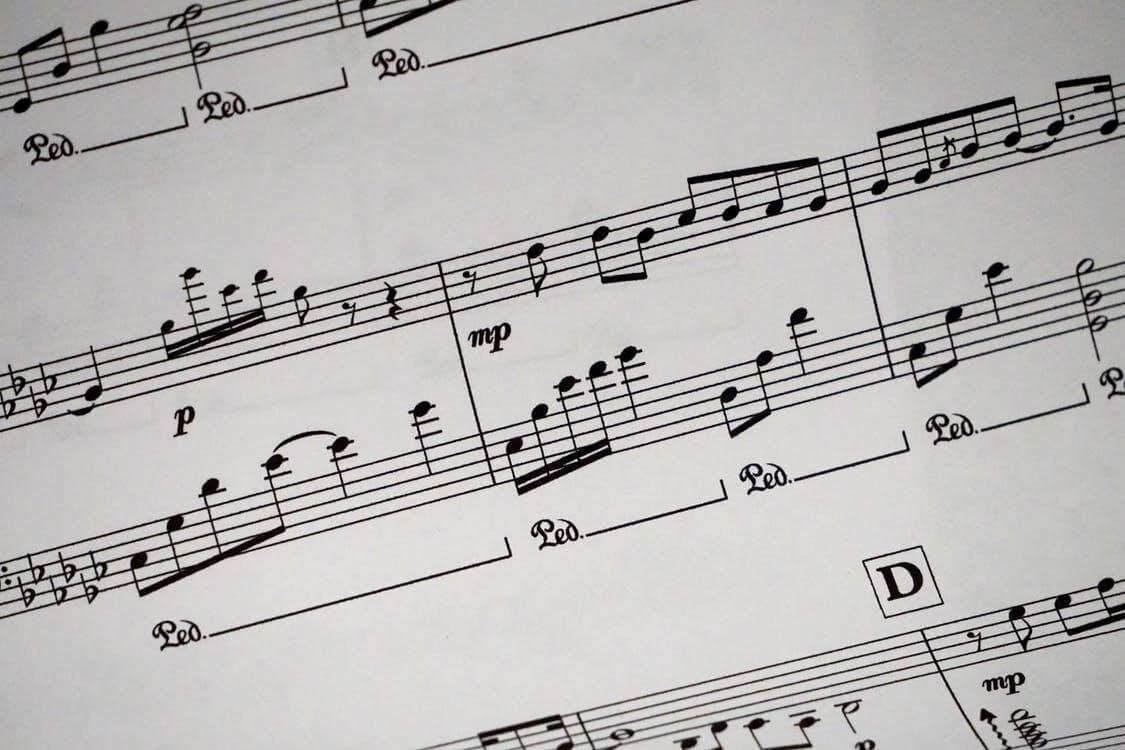My favorite application of the Mandelbrot set. As someone who writes music and is looking for interesting ways to write music, I am very interested in this topic.

You may wonder: how can fractals and mathematics in general, relate to music? And it turns out it can, in the most direct way. Pythagoras noticed that the ratio of frequencies of two neighboring notes is always different, but the ratio of frequencies of two notes, separated by four positions, on the contrary, is always constant and is 3/2. This consonance is now called a quint. Taking the quint as a basis, Pythagoras derived a musical formula, which allows you to use the frequency of the base note, from which you are counting, and the sequence number of a given note to get the desired value of the frequency of the next note. The successive application of the formula results in sounds that are one quint away from each other. This row contains all the notes of the sound scale. Even though they belong to different octaves, by dividing or multiplying the frequency of the desired sound by two, you can move it to the neighboring octave.

Repeating the operation of division (or multiplication) several times, you can fill the entire range of the instrument. The role of mathematics here is obvious. However, there is another side to this story: in the Pythagoras formula, the whole number of quintals does not fit into the whole number of octaves. This discrepancy is called the “Pythagorean comma”. The Pythagorean Comma is not only an apparent mathematical paradox.

Centuries later, the problem was solved by the German composer Andreas Werkmeister. Werckmeister created his own sound scale instead of the natural one, basing his system on three postulates:

• The ratio of the frequencies of the same notes in neighboring octaves must be two;
• Between these frequencies there must lie exactly twelve notes, according to the number of semitones in an octave;
• All semitones must be equal.

In accordance with these postulates, Werkmeister divided the octave into twelve absolutely equal semitones. Such a chord was called temperament. The essence of temperament consists in small changes in the value of intervals compared with their acoustically accurate value. In the 12-step evenly tempered formation all pure quintals are reduced by 1/12 of the Pythagorean comma. As a result, the system became closed, the octave was divided into 12 equal semitones and all the intervals of the same name became equal in magnitude.

What happened next? The union of mathematics and music actively continued to develop. In the 20th century, it is embodied in a new musical instrument, the computer, as well as in the established in the second half of the twentieth-century computer music, based on the theory of algorithms.

The practice of serialism prepared a fertile ground for the use of algorithms in electronic music. The sequential algorithmic method of composing music was first proposed by Josef Matthias Hauer (1883-1959). Hauer (and independently of Arnold Schoenberg) introduced such a technique of musical composition as dodecaphony.

Dodecaphony refers to the serial technique of constructing musical compositions. The serial technique involves using a series of unrepeated sounds as the pitch basis of a musical work. The main principle of dodecaphony theory is the inadmissibility of repeating the same sounds in time, until all 12 sounds, into which an octave is divided within a tempered system, are exhausted. The sequence of 12 non-repeating sounds forms a series. The series is the basic element of the entire musical composition. The sounds of the series form consonances and some of the sounds may form a melody. The basic law is the prohibition of changing the sequence of sounds in a series, breaking the order of their sequence. There are varieties of series: rakohod (don’t laugh, it is just a series read from the last sound to the first one), inversion (a series obtained from the original sequence by replacing all intervals with their reversals), and rakohod inversion (coincides with rakohod inversion with the accuracy of transposition). Thus, thanks to the rules formulated in advance, it is possible to determine the whole composition and its particulars (macro- and microform) and Hauer’s method can be considered a precursor of modern computer technology in this field.

Examples of dodecaphony can be seen in Figure 8 and Figure 9.

Fig. 8 A. Schoenberg. Quintet for Wind Instruments Op. 26 (Excerpt)

Fig. 9 A. Berg. Concerto for violin and orchestra. (Excerpt)

А. Vasiliev, the author of the article “Music Composer Based on Multilayer Neural Network,” singles out as one of the types of algorithmic composition, the composition based on the application of mathematical functions, including fractals.

In the ’70-’80s, the study of the behavior of systems of nonlinear dynamic equations aroused interest in their use in algorithmic composition (Pressing, DiScipio, Gogins, Bidlack, Leach), and today in the musical art one of the most popular methods of creating music based on algorithms is the method of the so-called fractal composition.

Thus, the use of fractals in the musical art and science occupies an important place. This research is based on the theory of Richard Voss, who established that any sound has fractal properties. The researcher identified three categories of sound based on mathematical elements:

1. white noise – random noise, defined as disturbing to the listener (Fig.10)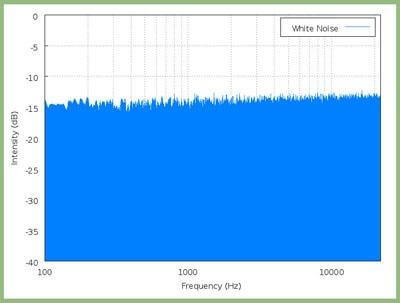Fig. 10

2. pink noise – occupies an intermediate position, more structured than white noise, it is the most pleasant for the listener’s perception (Fig.11);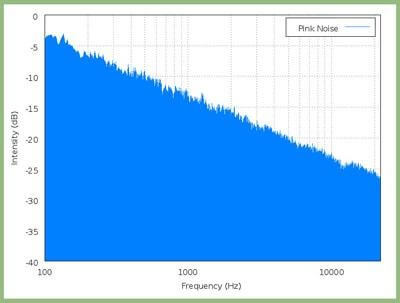Fig. 11

3. brown noise – structured noise, defined as mechanical for the listener (Fig.12).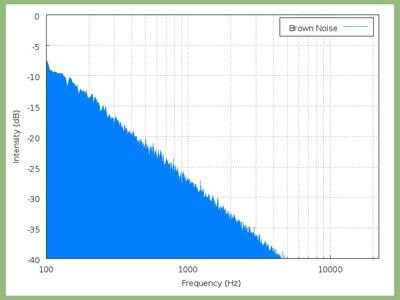Fig. 12

Р. Voss discovered that by exploring the fractal nature of pink noise, it is possible to generate melodies pleasing to the ear, as pink noise is a balance between complete chaoticity and extreme structuredness. The research of Martin Gardner, Michael Schroeder, Jerry Chesnut, Stewart Mason, and Max Saffley is aimed at studying the fractal nature of music. For example, Leonid Solomon – the author of “The Fractal Nature of Music” associates Sierpinski’s triangle with the rondo form, and on the basis of the Koch snowflake triangle generates polyphonic works. The goal of all these studies is to find something universal in musical works, something that stands above individual cultures and artistic taste.

Thus, our considered approaches to the use of fractals in music indicate that modern science offers new methods to study not only the phenomena of nature but also art. Modern researchers on the basis of the proposed methods are trying to understand music as abstract art, as well as to identify some universal laws of creating a musical work. These problems of musical creativity and science have worried scientists and musicians for many centuries, and the 21st century has made its contribution based on objective mathematical language.

So, I have made this short experimental album using fractal music methods to see everything in practice. I used:

• FractMus 2000
• Fl Studio + VST Plugins

You are welcome to listen to it here: Indigo Fractal Of The Ankh Box by NoMan.exe | No Man Exe | Free Listening on SoundCloud

##### Visual application of the Mandelbrot set

The pictures of astonishing beauty made Mandelbrot’s set famous. But, color in this case is more of an artistic device than something deeply scientific. To understand the nature of color, let’s first color Mandelbrot’s multitude in shades of gray as follows. Let’s define some numbers for the grayscale range. It is convenient to choose 256 gradations, and number them as follows: 0 – white, 255 – black. Then, we take a point and substitute it in the iterative sequence. If it flies away (at the zero-step), color it white. If it’s at the first step, let’s paint it “a little bit gray”. If say, at 128th, the dot will have a saturated (50%) gray color. If at 254th, it is almost black.

And if the point gets to the 255th step, we will consider that it belongs to the Mandelbrot set, and we will paint it black.

So, we get a grayscale picture, which, generally speaking, makes some sense. Here the color actually shows the speed at which the dot is fleeing. The brighter the dot, the faster it flies away. The rest is pure art. You can make gradations of any color instead of grayscale. You can define a palette with any number of colors and assign a color to a point according to the principle described above – the fleeing step is equal to the color number in the palette (Fig.13).

Fig. 13 The Mandelbrot set in shades of gray

Finding beautiful images of Mandelbrot sets is an interesting hobby for very many people. They collect such images, and each of them can be described by a small number of parameters, for example, just coordinates of the center.

There are plenty of programs for drawing fractals, but even so, many people write their own programs for more flexibility when experimenting.

#### My application

I stumbled upon my program while examining the contents of my old hard drives. I was lucky enough to have not only the program itself, but also its source code, which I will also provide a link to.

When I was a schooler, I decided to apply the Mandelbrot set not only to music but also to programming. Armed with a huge C++ book and information on the Internet I started creating a program that would draw fractal images.

I did it using C++ Builder. No, I wasn’t a genius who learned C++ at a high level at school age and could program anything. Yes, my knowledge of C++ was at the level of basic syntax. Using books and the internet and after many retries and annoying errors, I have created my own program.

My program performed the following functions:

1. visualization of the result of the Mandelbrot set when you change the following conditions:

• choice of color palette;
• changing the number of iterations in calculations;
• consecutive double increase/decrease of user-selected fragment of set.
2. visualization of behavior of set when the degree, in which a complex number is raised, changes;

Figure 14 shows the appearance of the start window of the program.

Fig. 14 Start window of the program

In the simplest case, to construct a black-and-white Mandelbrot set we need to choose:

• the maximum number of iterations of a point at which we will assume that it belongs to the set;
• the area in the plane in which we are going to search for points of the set.

A 4 × 4 square with the center at the origin of the coordinates is chosen to represent the whole set. The area on the plane corresponds to a window on the screen. It is convenient to proceed as follows: go through the points (pixels) of the window, for each point find its coordinates on the plane, and then start the iterative process.

The dependence of the appearance of the set on the number of iterations can be clearly seen in Figs. 15 and 16.

Fig. 15 Mandelbrot set at 5 iterations

Fig. 16 The Mandelbrot set at 200 iterations

The dependence of the appearance of the set on the choice of color palette is demonstrated in Fig. 17, 18. These drawings show the same area of the coordinate plane with 128x magnification.

Fig. 17 The Mandelbrot set in shades of gray

Fig. 18 The Mandelbrot set in color

The dependence of the appearance of the set on the degree to which a complex number is erected is shown in Figs. 19, 20, 21.

Fig. 19 The Mandelbrot set of the third degree in the black and white palette (Mandelbrot bulb)

Fig. 20 The Mandelbrot set of the fourth degree in the black and white palette

Fig. 21 The Mandelbrot set of the 16th degree in the chess palette

You are welcomed to test my application! You can download it here. No Linux/Mac version, sorry 🙂

Here’s a small part of source code with some comments.

You can also see a video recording of how the application works

#### Conclusions

From the educational point of view, projects related to the construction of the Mandelbrot set has advantages:

• They are quite easy to implement (at a basic level);
• They are very beautiful (at any level!).

But there are also difficulties, the true mathematics associated with the Mandelbrot set definitely lies outside the basic math knowledge, so a number of important things have to be learned.

Thank you for reading my article! Hope it was interesting.

I will try to tell you more interesting things in the near future, so stay tuned 😉

#### Resources##### Connect with us
Bulgara Street 33/1, Chisinau MD-2001, Moldova
+ 373 22 996 170
info@isd-soft.com
De Amfoor 15, 5807 GW Venray-Oostrum, The Netherlands
+ 31 478 502944